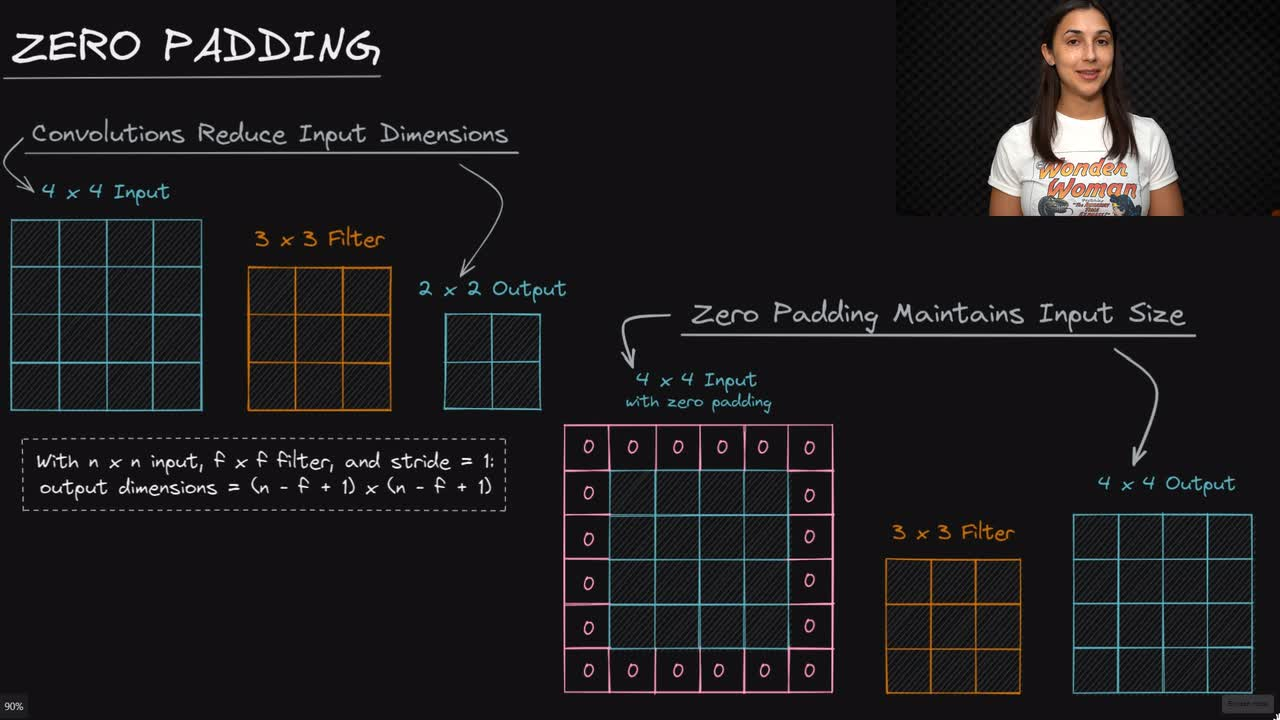# Deep Learning Dictionary - Lightweight Crash Course

Deep Learning Course - Level: Beginner

## Zero Padding for Neural Networks - Deep Learning Dictionary

### video

locklock

### Zero Padding - Deep Learning Dictionary

Convolutional layers in a CNN have a defined number of filters that convolve the image input, and as a result, the output from the convolutional layers have reduced dimensions from the original image input.

We have a general formula for determining the dimensions of the convolved output. Assuming a stride of $$1$$, if our image is of size $$n \times n$$, and we convolve it with an $$f \times f$$ filter, then the dimensions of the resulting output is

lock

### resources

lock

lockDEEPLIZARD Message notifications